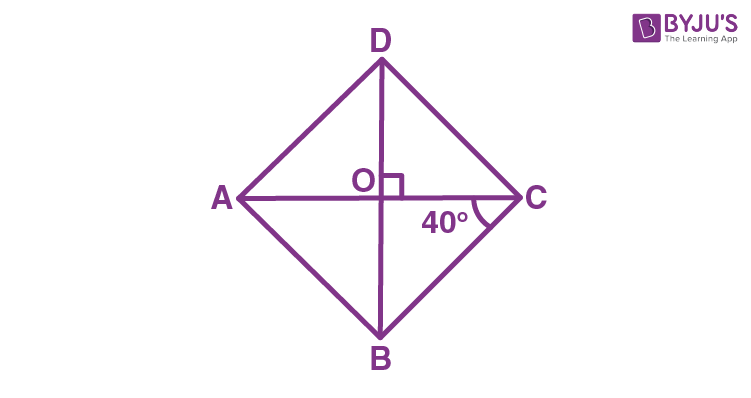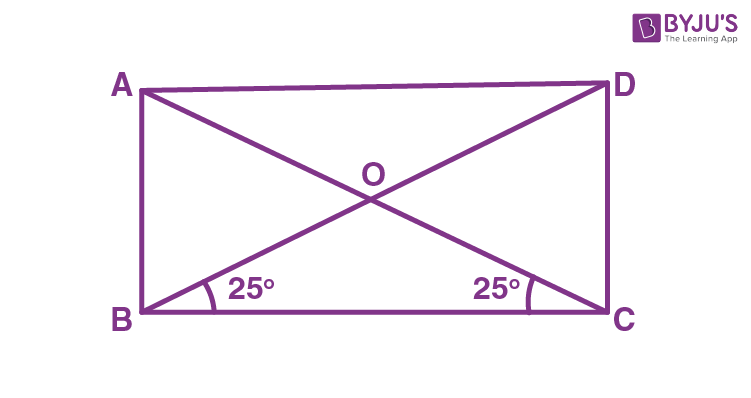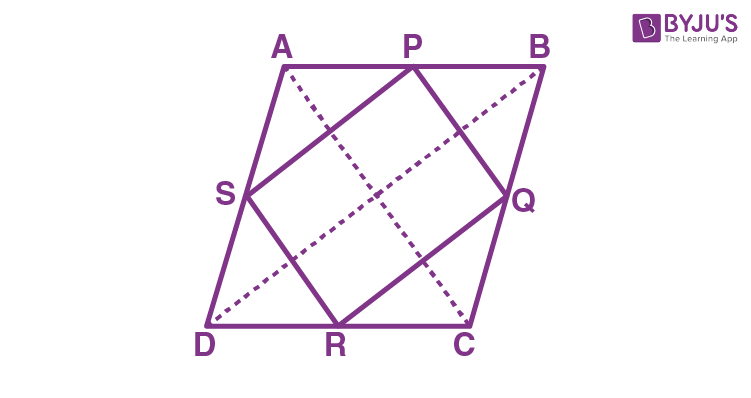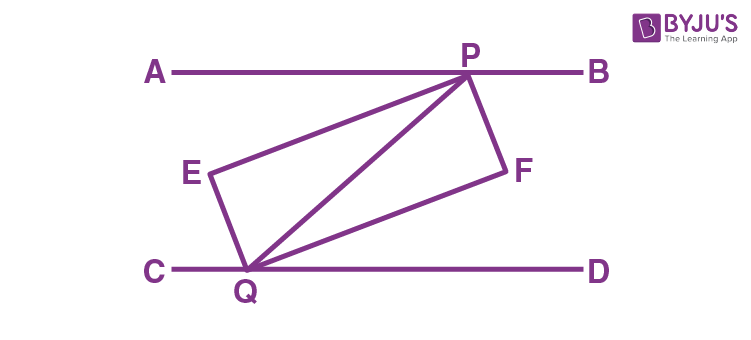# Class 9 Maths Chapter 8 Quadrilaterals MCQs

Class 9 Maths Chapter 8 Quadrilaterals MCQs are available here with answers, online. The questions are prepared, as per the CBSE syllabus and NCERT curriculum. Students can prepare for exams with the help of these objective questions to score good marks. The answers are available with detailed explanations. Get chapter-wise MCQs at BYJU’S and also check Important Questions for Class 9 Maths.

## MCQs on Class 9 Maths Chapter 8 Quadrilaterals

Solve the questions provided below with four multiple options and choose the correct one.

1) The quadrilateral whose all its sides are equal and angles are equal to 90 degrees, it is called:

a. Rectangle

b. Square

c. Kite

d. Parallelogram

2) The sum of all the angles of a quadrilateral is equal to:

a. 180°

b. 270°

c. 360°

d. 90°

3) A trapezium has:

a. One pair of opposite sides parallel

b. Two pairs of opposite sides parallel to each other

c. All its sides are equal

d. All angles are equal

Explanation: A trapezium has only one pair of opposite sides parallel to each other, and the other two sides are non-parallel.

4) A rhombus can be a:

a. Parallelogram

b. Trapezium

c. Kite

d. Square

5) A diagonal of a parallelogram divides it into two congruent:

a. Square

b. Parallelogram

c. Triangles

d. Rectangle

6) In a parallelogram, opposite angles are:

a. Equal

b. Unequal

c. Cannot be determined

d. None of the above

7) The diagonals of a parallelogram:

a. Equal

b. Unequal

c. Bisect each other

d. Have no relation

8) Each angle of the rectangle is:

a. More than 90°

b. Less than 90°

c. Equal to 90°

d. Equal to 45°

Explanation: Let ABCD is a rectangle, and ∠A = 90°

AD || BC and AB is a transversal

∠ A + ∠ B = 180° (Interior angles on the same side of the transversal)

∠ A = 90°

So, ∠ B = 180° – ∠ A = 180° – 90° = 90°

Now, ∠ C = ∠ A and ∠ D = ∠ B (Opposite angles of the parallelogram)

So, ∠ C = 90° and ∠ D = 90°

Hence all sides are equals to 90°.

9) The angles of a quadrilateral are in the ratio 4: 5: 10: 11. The angles are:

a. 36°, 60°, 108°, 156°

b. 48°, 60°, 120°, 132°

c. 52°, 60°, 122°, 126°

d. 60°, 60°, 120°, 120°

Explanation: Let x be the common angle among all the four angles of a quadrilateral.

As per angle sum property, we know:

4x+5x+10x+11x = 360°

30x = 360°

x = 12°

Hence, angles are

4x = 4 (12) = 48°

5x = 5 (12) = 60°

10x = 10 (12) = 120°

11x = 11 (12) = 132°

10) If ABCD is a trapezium in which AB || CD and AD = BC, then:

a. ∠A = ∠B

b. ∠A > ∠B

c. ∠A < ∠B

d. None of the above

Explanation: Draw a line through C parallel to DA intersecting AB produced at E.

BC = CE

⇒ ∠CBE = ∠CEB

also,

∠A + ∠CBE = 180° (Angles on the same side of transversal and ∠CBE = ∠CEB)

∠B + ∠CBE = 180° ( As Linear pair)

⇒ ∠A = ∠B

11) Which of the following is not true for a parallelogram?

(a) Opposite sides are equal

(b) Opposite angles are equal

(c) Opposite angles are bisected by the diagonals

(d) Diagonals bisect each other.

Explanation: Opposite angles are bisected by the diagonals is not true for a parallelogram. Whereas opposite sides are equal, opposite angles are equals, diagonals bisect each other are the properties of a parallelogram.

12) Three angles of a quadrilateral are 75º, 90º and 75º. The fourth angle is

(a) 90º

(b) 95º

(c) 105º

(d) 120º

Explanation: We know that the sum of angles of a quadrilateral is 360º.

Let the unknown angle be x.

Therefore, 75º+90º+75º+x = 360º

x = 360º – 240º = 120º.

13) ABCD is a rhombus such that ∠ACB = 40º. Then ∠ADB is

(a) 40º

(b) 45º

(c) 50º

(d) 60º

Explanation: We know that the diagonals of the rhombus bisect each other perpendicularly.By using the alternate interior angles, and angle sum property of triangle, we can say:

From the triangle, BOC,

∠BOC + ∠OCB + ∠OBC = 180º

(where  ∠BOC= 90º, ∠OCB = 40º)

90º+40º+ ∠OBC = 180º

∠OBC = 180º – 130º

∠OBC = 50º

∠OBC =∠DBC

Now, by using alternate angles, we can say

14) The quadrilateral formed by joining the mid-points of the sides of a quadrilateral PQRS, taken in order, is a rhombus, if

(a) PQRS is a rhombus

(b) PQRS is a parallelogram

(c) Diagonals of PQRS are perpendicular

(d) Diagonals of PQRS are equal.

Explanation: The quadrilateral formed by joining the mid-points of the sides of a quadrilateral PQRS, taken in order, is a rhombus if the diagonals of PQRS are equal.

15) A diagonal of a rectangle is inclined to one side of the rectangle at 25º. The acute angle between the diagonals is

(a) 25º

(b) 40º

(c) 50º

(d) 55º

Explanation: Consider the rectangle ABCDIn a triangle BOC,

∠OBC = ∠OCB (Opposite angles of isosceles triangle)

Therefore, ∠OBC + ∠OCB+∠BOC = 180º

25º+25º + ∠BOC = 180º

∠BOC = 180º- 50º

∠BOC = 130º.

By using the linear pair,

∠AOB + ∠BOC = 180º

∠AOB = 180º – 130º

∠AOB= 50º

Hence, the acute angle between the diagonals is 50º.

16) If angles A, B, C and D of the quadrilateral ABCD, taken in order, are in the ratio 3:7:6:4, then ABCD is a

(a) Kite

(b) Rhombus

(c) Parallelogram

(d) Trapezium

Explanation:

Given that, the angles A, B, C and D of a quadrilateral are in the ratio 3:7:6:4.

We know that A+B+C+D = 360º

Hence, now we can assume, 3k+7k+6k+4k= 360º

20k = 360º

k=18º

Therefore, A = 3k = 54º

B= 7k = 126º

C = 6k = 108º

D = 4k= 72º

If we draw the quadrilateral with these angles, we get a trapezium.

17) The quadrilateral formed by joining the mid-points of the sides of a quadrilateral PQRS, taken in order, is a rectangle, if

(a) PQRS is a rectangle

(b) PQRS is a parallelogram

(c) Diagonals of PQRS are perpendicular

(d) Diagonals of PQRS are equal

Explanation: The quadrilateral formed by joining the mid-points of the sides of a quadrilateral PQRS, taken in order, is a rectangle, if the diagonals of PQRS are perpendicular.

18) The figure obtained by joining the mid-points of the sides of a rhombus, taken in order, is

(a) a square

(b) a rhombus

(c) a rectangle

(d) any parallelogram

Explanation: Let ABCD be a rhombus. P, Q, R, S be the midpoint of the sides AB, BC, CD and DA. If we join the midpoints, we will get the shape rectangle.19) If APB and CQD are two parallel lines, then the bisectors of the angles APQ, BPQ, CQP and PQD form

(a) Square

(b) Rectangle

(c) Rhombus

(d) any other parallelogram

Explanation:Hence, the bisectors of the angles APQ, BPQ, CQP and PQD form the shape rectangle.

20) Which of the following is not a quadrilateral?

(a) Kite

(b) Square

(c) Triangle

(d) Rhombus

Explanation: square, kite and rhombus are quadrilaterals as it has four sides. Whereas a triangle is not a quadrilateral as it has only three sides.

Stay tuned with BYJU’S – The Learning App and download the app today to get more class-wise concepts.

1. Hii,thank you for your questions I think it will help me in my exam. Wish me good luck 🥰🥰

1. byus is the best learning app always

2. Arpan pandey

Thanks for giving a nice mcq type questions and again thanku

2. thanks you for these question . byjus is the best app for learning

3. Gyandeep

I am learning from byjus app
I am loved this app

4. nice questions and easy too!

5. Thank you byjus for an helpful MCQ type questions. Tomorrow is the my exam and I am now well prepared